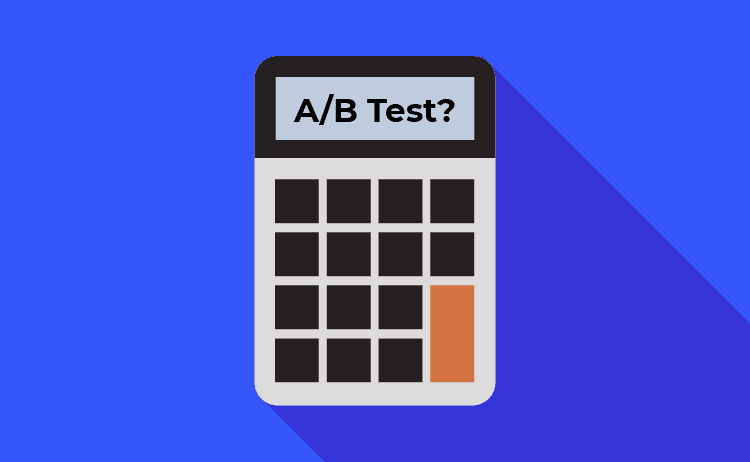# The ASO A/B Test Bluff: Why You Pay Less and Lose More

Life is riddled with various dilemmas one faces when forced to make decisions. For App Store Optimization (ASO) specialists, we are perpetually faced with the decision of which creatives to choose for our app stores in order to improve app install conversion rates. An immediate solution to this conundrum is to A/B test a myriad of creatives and choose the one that converts better than most.

But what goes unspoken is that it’s really not that simple, nor always accurate. And it’s what I like to call the A/B testing bluff. As much as we all love to flirt with the notion of eliminating the guesswork in our ASO decision-making, some of the most popular A/B testing methods and tools are actually grossly inaccurate.

For example, A/B testing tools and calculators are inexpensive (or even free), easy-to-use, and therefore exceedingly popular in our industry. However, many of these calculators are prone to statistical errors that produce invalid results. Such errors are most often caused by what statisticians call post-hoc theorizing, which fundamentally distorts the results of statistical analyses.

I’ll do my best to help you better understand the A/B testing process, where popular A/B testing calculators get it wrong, and I’ll provide you with information on how StoreIQ™ has proven to be the most statistically sound and effective way to test app store creatives.

# The A/B testing process

When practicing statistics, most professionals apply the common statistical method of hypothesis testing. In terms of ASO, for example, hypothesis testing can be used to determine if there is a difference between two different app store creatives in relation to CVR.

There are four key stages to A/B testing.

## 1. Define the Null Hypothesis

The testing process begins with a null hypothesis, which is essentially a declaration about the data that we would like to refute. Since we want to find out if there’s a difference between two groups, we would like to refute the notion that there is no difference.

In the example of testing two different app store creatives, we are looking to refute the notion that there is no difference in CVR between the two creatives.

The Null Hypothesis therefore states that there is no difference in CVR for creative a and creative b.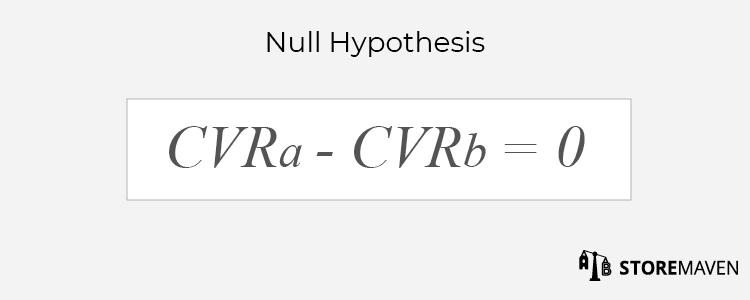Hence, the alternative to the null hypothesis is that there is a difference in CVR for creative a and creative b.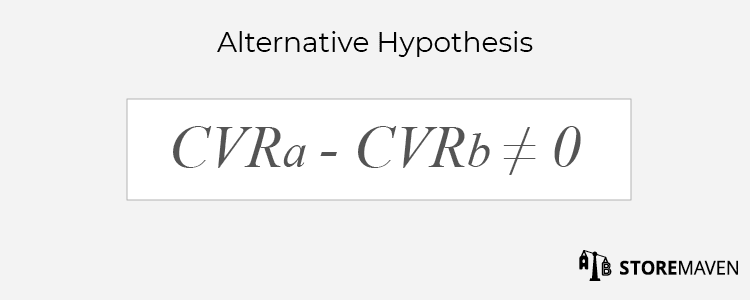This hypothesis set is referred to as a two-sided test.

## 2. Design Experiment

The next step is to design the experiment. There are various statistical factors that must be taken into consideration when A/B testing creatives. Here are some important factors that need to be considered in the design stage:

• Is the sample representative of the target population?
• What is the minimum sample size?
• How do we properly randomize variations users are served?
• What factors can impact the results (i.e., time of day, day of week, etcetera)?
• What is a meaningful difference in creative variations?

These factors are not only pertinent for successful experiment design but they are imperative for valid testing.

## 3. Gather Data

After you design your experiment, it’s time to gather data. Gathering data involves using a platform that can present different creatives you would like to A/B test to users from a wide range of targeting and traffic sources.

## 4. Analyze Data

Once we have gathered data, we apply the statistical formula that concludes whether the results are significant or not. Significance is a measurement of the probability that the null hypothesis is true. Since our aim in hypothesis testing is to refute the null hypothesis, then this measurement should be low (e.g., 5% significance level is the most common threshold used for hypothesis testing, it can also be referred to as 95% confidence level).

## Limitations of A/B testing in ASO

There are several limitations that you face when applying the traditional A/B testing process to ASO.

A major limitation has to do with the fact that A/B testing results can be volatile when testing over time. That is, the variation ranking can change frequently, even on a daily basis. In this case, you may reach the conclusion that on the first day variation a won, though if you waited another day you would see that variation b actually performs better. Implementing the wrong variation based on this test could cost you the added users that would install had they been exposed to the better variation. The problem is that the traditional A/B testing process entirely disregards periodic variations in data.

Furthermore, when you test two variations that are similar to each other, the difference in CVRs are typically extremely small. In these cases, it is impossible to reach significance when using small sample sizes. Therefore, this setting requires a very large sample size, which is costly when using a sandbox solution.

# Where Popular A/B Testing Calculators Really Get It Wrong

The biggest problem many app developers experience when utilizing A/B testing tools, is after applying winning variations they don’t see real store results. This is a direct result of the fact that most A/B testing tools rely on the wrong formula.

This formula is based off the way in which calculators approach the A/B testing process. Instead of starting the process by defining the hypothesis, they observe the results of an A/B test first and then define the hypothesis based on what they observed. This process is referred to as post-hoc theorizing, or Hypothesizing After the Results are Known (HARKing), which is “widely seen as inappropriate” in the scientific community.

In our creative A/B test example, the formulaic definition of this type of hypothesis is defined as the creative with the larger CVR is superior to the other creative. This hypothesis is referred to as a one-sided test.

Note the difference: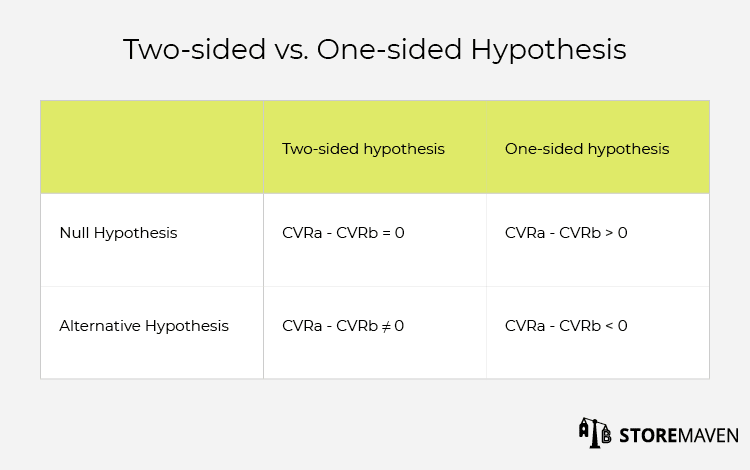The premise of an A/B test should be that CVRs are random variables, that way the observations can be treated as random results of a statistical process. Once we have the data in front of us, and we base our hypothesis on that data, we minimize the inherent randomness of the statistical test setting. Since we have no way of knowing which creative is superior without testing, our hypothesis must be that they are different and not that one is better than the other.

Subsequently what happens in post-hoc theorizing is that you end up using a formula that will always give a lower significance, which leads to a higher probability of falsely rejecting the null hypothesis.

Here is an example of how this pseudo-methodology can lead to erroneous results.

Let’s say you are testing two different Feature Graphics…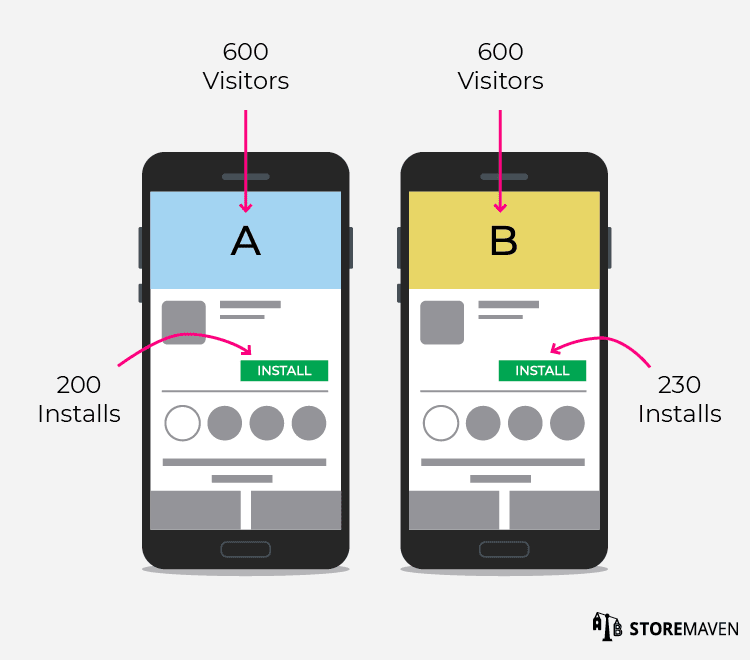Again, we perform our analyses using the commonly used significance level of 5% (95% confidence level). Now let’s say that 600 visitors are exposed to each Feature Graphic. Feature Graphic a gets 200 installs and Feature Graphic b gets 230 installs.

If we employ the traditional A/B testing process, then the null hypothesis would be that Feature Graphic a and Feature Graphic b have the same CVR. Hence the alternative hypothesis is that they are not equal.

If we then plug the data above into the widely used proportion difference test formula, we get a non-significant p-value. (The p-value is the probability that the alternative hypothesis is incorrect.)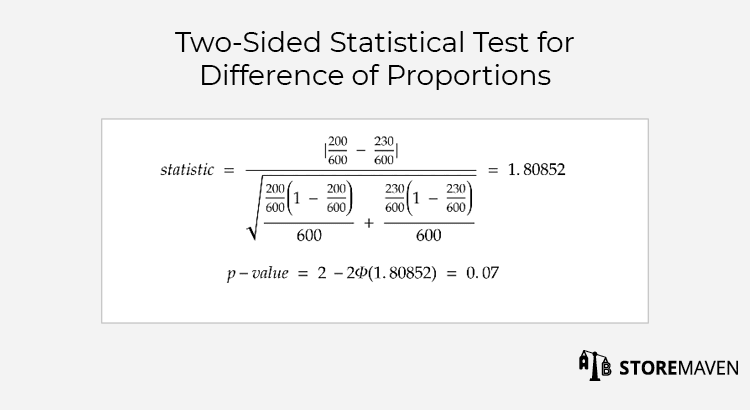Notice how the test p-value for this setting is 0.07, which is larger than the significance level of 5%.

If we use the post-hoc theorizing approach and apply the same exact data to the formula, then we get precisely half of the value of the significance.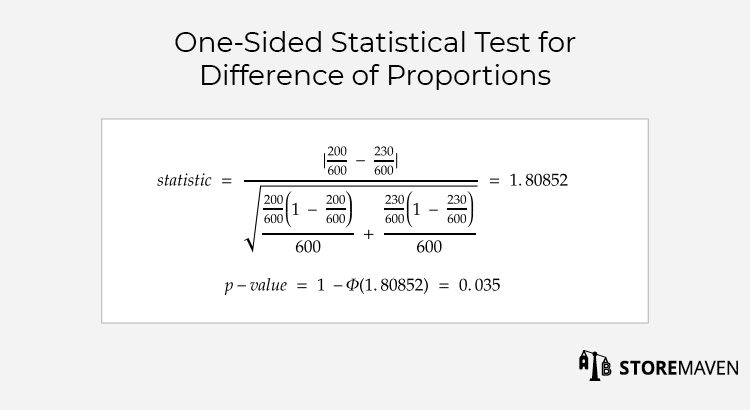This prompts us to wrongly reject the null hypothesis, since the p-value is now lower than the significance level.

Hence, in addition to the inherent limitations of applying the traditional A/B testing process to ASO that were previously listed, popular calculators further get it wrong in calculations they use in the testing process.

# StoreIQ™ as the optimal testing solution

Backed by big data*, we invented StoreIQ™ to help us detect patterns and advance app store creative testing as a scientific method that yields real store results. StoreIQ™ is a proprietary, predictive algorithm that estimates the CVR of test variations quickly and far more accurately than standard A/B testing methodologies or calculators.

The required sample size for StoreIQ™ is optimized to deliver a highly accurate result while keeping the sample size small. Our algorithm predicts when a variation will underperform, and it stops the variation immediately so that traffic spend is not wasted on a variation that is not likely to win. This saves our clients 30-50% on the cost of sending traffic to a test, while maintaining accurate results. This is proven by real store results.

Furthermore, since there are numerous outside variables that can affect the experiment, StoreIQ™ also evolves as the test progresses and reacts to changes in external factors contrary to the static A/B test approach. Such factors include, but are not limited to, periodic variations in data when testing over time and time of day.

Although there is much more to be said on the subject, I hope you now understand the fundamentals of common A/B testing inaccuracies and how StoreIQ™ is the optimal solution to accurately and cost-effectively test app store creatives. Our account managers will be happy to further explain the advantages of using the StoreMaven platform or answer any high-level questions you may have. Feel free to reach out at info@storemaven.com!

*Our company’s datasets include more than 1 billion data points dating back four years.